Domination

Domination

Domination是一個泛稱，專指「支配鄰近元件」這一類的圖論主題，例如Packing與Covering。

「填裝Packing」是使用一種元件，填滿圖上全部的點、或者邊。元件用量越多越好。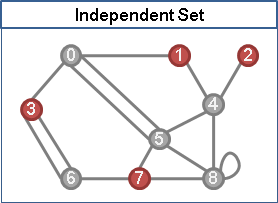「覆蓋Covering」是使用一種元件，蓋住圖上全部的點、或者邊。元件用量越少越好。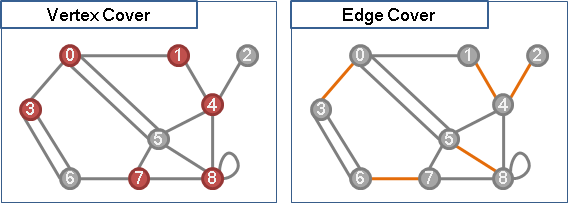Independent Set

Independent Set```Maximum Independent Set [NP-complete]

Maximum Independent Set in Tree [P]

Maximum Independent Set in Bipartite Graph [P]

```

UVa 193 11065 11069 1220

Independent Edge Set（Matching）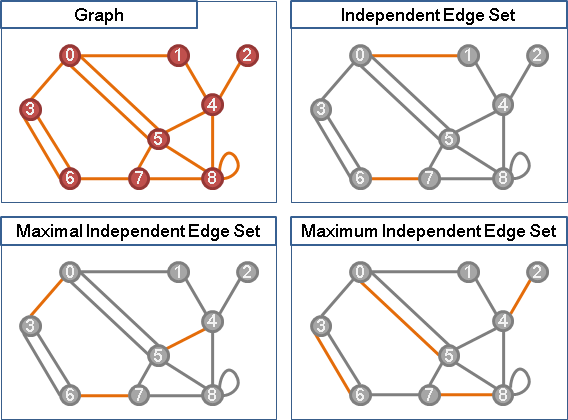Maximum Independent Set

Maximum Independent Set in Tree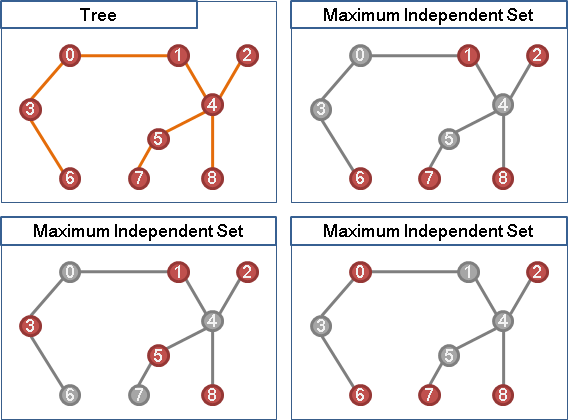```一、樹葉與父親都是最大獨立集：不成立。

```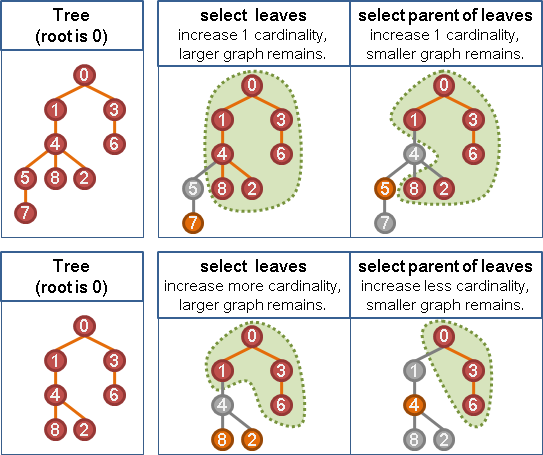Greedy Method。由樹葉往樹根方向選出獨立集，儘量選擇樹葉，最後就得到最大獨立集。不過這種方式無法得到字典順序最小的最大獨立集。

```一、建立DFS Tree，找出preorder。

```
```一、建立BFS tree，找出levelorder。

```

Maximum Independent Set in Bipartite Graph```甲、X側未匹配點的交錯樹們。

```

Dominating Set

Dominating Set```Minimum Dominating Set [NP-complete]

Minimum Dominating Set in Tree [P]

Minimum Dominating Set in Bipartite Graph [NP-complete]

```

UVa 10160 1218

Edge Dominating Set

```Minimum Edge Dominating Set [NP-hard]

```

Independent Set與Dominating Set

```independent set

dominating set

```
```maximal independent set

maximum independent set

```「獨立支配集」。既是支配集、又是獨立集。

Maximal Irredundant Set = Minimal Dominating Set，一一對應。

Vertex Cover

Vertex Cover```Minimum Vertex Cover [NP-complete]

Minimum Vertex Cover in Tree [P]

Minimum Vertex Cover in Bipartite Graph [P]

```

UVa 10243 10859 10984 11419 11095 ICPC 2897

Edge Cover

Edge Cover```Minimum Edge Cover [P]

Minimum Edge Cover in Bipartite Graph [P]

Minimum/Maximum Weight Edge Cover [P]

```

UVa 10349

Minimum Edge Cover

Packing與Covering

```General Graph:

|Maximum Independent Set|      + |Minimum Vertex Cover| = |V|
|Maximum Independent Edge Set| + |Minimum Edge Cover|   = |V|

```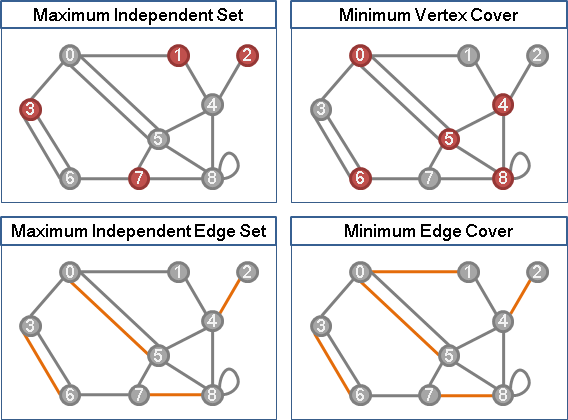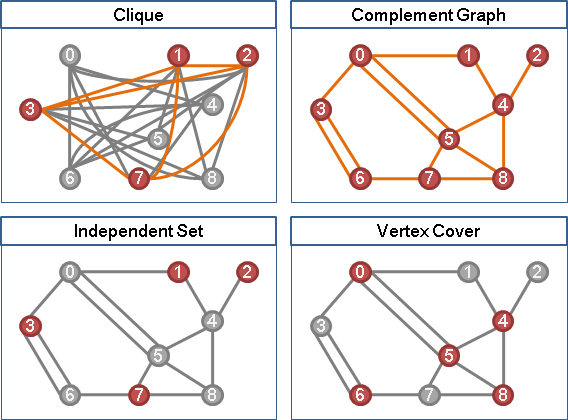Packing與Covering in Bipartite Graph

```Bipartite Graph:

|Maximum Independent Set|       = |Minimum Edge Cover|
|Maximum Independent Edge Set|  = |Minimum Vertex Cover|

|Maximum Independent Set|      + |Minimum Vertex Cover| = |V|
+                             +
|Maximum Independent Edge Set| + |Minimum Edge Cover|   = |V|
||                            ||
|V|                           |V|
```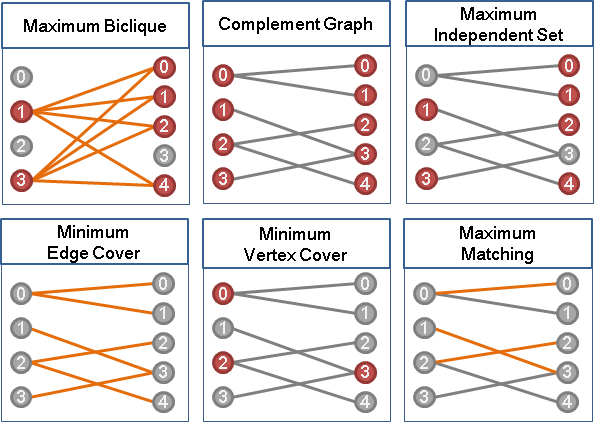UVa 11159 12083 12168 ICPC 6309

Path Domination

```問題有兩種：Packing、Covering。

```

Packing系列

```{Minimum Cardinality} Vertex-disjoint Path + Vertex + Packing
Graph [NP-hard] 等同許多條Hamilton Path。
Tree  [Linear]  Greedy Method。從樹葉往樹根方向選出路徑。
建立BFS Tree。
以levelorder的逆序拜訪各點。
如果該點的鄰邊超過二條，
就隨意留下兩條連往小孩的邊，刪除其餘鄰邊。
DAG   [P]       化作Maximum Cardinality Bipartite Matching。
DAG的邊i->j，對應到二分圖的邊Xi->Yj。
當匹配數越大，則末端端點越少，則路徑數也越少。
```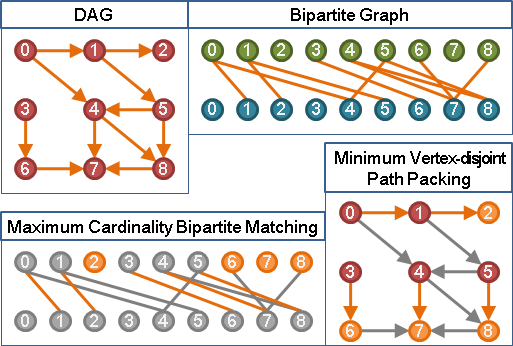UVa 11381 12831

```{Maximum/Minimum Weight} Vertex-disjoint Path + Vertex + Packing
Graph [NP-hard] 等同許多條Hamilton Path。
Tree  [P]       Dynamic Programming。從樹葉往樹根方向遞歸。
DAG   [P]       化作Maximum Weight Bipartite Matching。
```

ICPC 4141

```{Minimum/Maximum Weight} Vertex-disjoint Cycle + Vertex + Packing
Graph   [P]     即是Minimum/Maximum Weight 2-Factor。
Digraph [P]     化作Maximum/Minimum Weight Perfect Bipartite Matching。
DAG的邊i->j，對應到二分圖的邊Xi->Yj。
```ICPC 3353 7463

```{Minimum Cardinality} Edge-disjoint Path + Edge + Packing
Graph [P]       等同許多條Euler Path。
無向圖：不斷以奇點作為路徑起點。答案為奇點數目的一半。
有向圖：出邊多於入邊的點，走向入邊多於出邊的點。
```

UVa 10248

```{Minimum/Maximum Weight} Edge-disjoint Path + Edge + Packing
Graph [P]      其實就是整張圖所有邊的權重總和。trivial。
```
```{Minimum/Maximum Weight} Edge-disjoint Cycle + Edge + Packing
Graph [P]      Minimum Cost Flow。
```

ICPC 4030

Covering系列

```{Minimum Cardinality} Vertex-disjoint Path + Edge + Covering
Graph [P]      Minimum s-t Flow。源點連至沒有入邊的點，沒有出邊的點連至匯點。
```

UVa 1440 ICPC 4597